# Frank Solutions for Class 10 Maths Chapter 15 Similarity

Frank Solutions for Class 10 Maths Chapter 15 Similarity are available here. At BYJU’S, we have a team of expert faculty trying their best to provide exercise-wise solutions to students according to their level of understanding. Frank Solutions for Class 10 Maths Chapter 15 can be used as a mode of reference by the students to improve their conceptual knowledge and understand the different ways used to solve problems.

Chapter 15 – Similarity are figures having the same shape with corresponding sides proportional and corresponding angles equal. Students can learn different types of problems provided in this chapter on similarity by referring to Frank Solutions for Class 10 Maths. Click on the link given below to download the free PDF.

## Download the PDF of Frank Solutions for Class 10 Maths Chapter 15 Similarity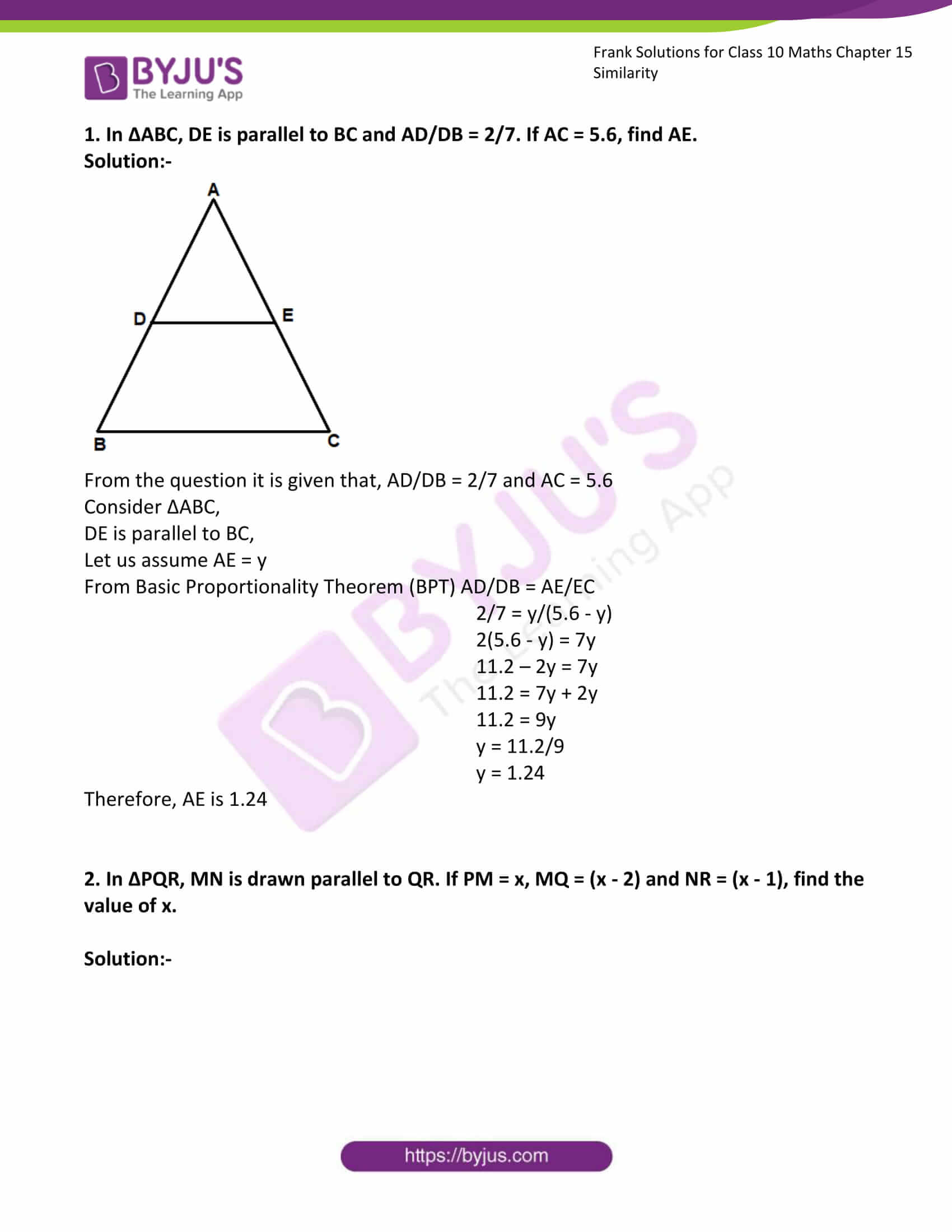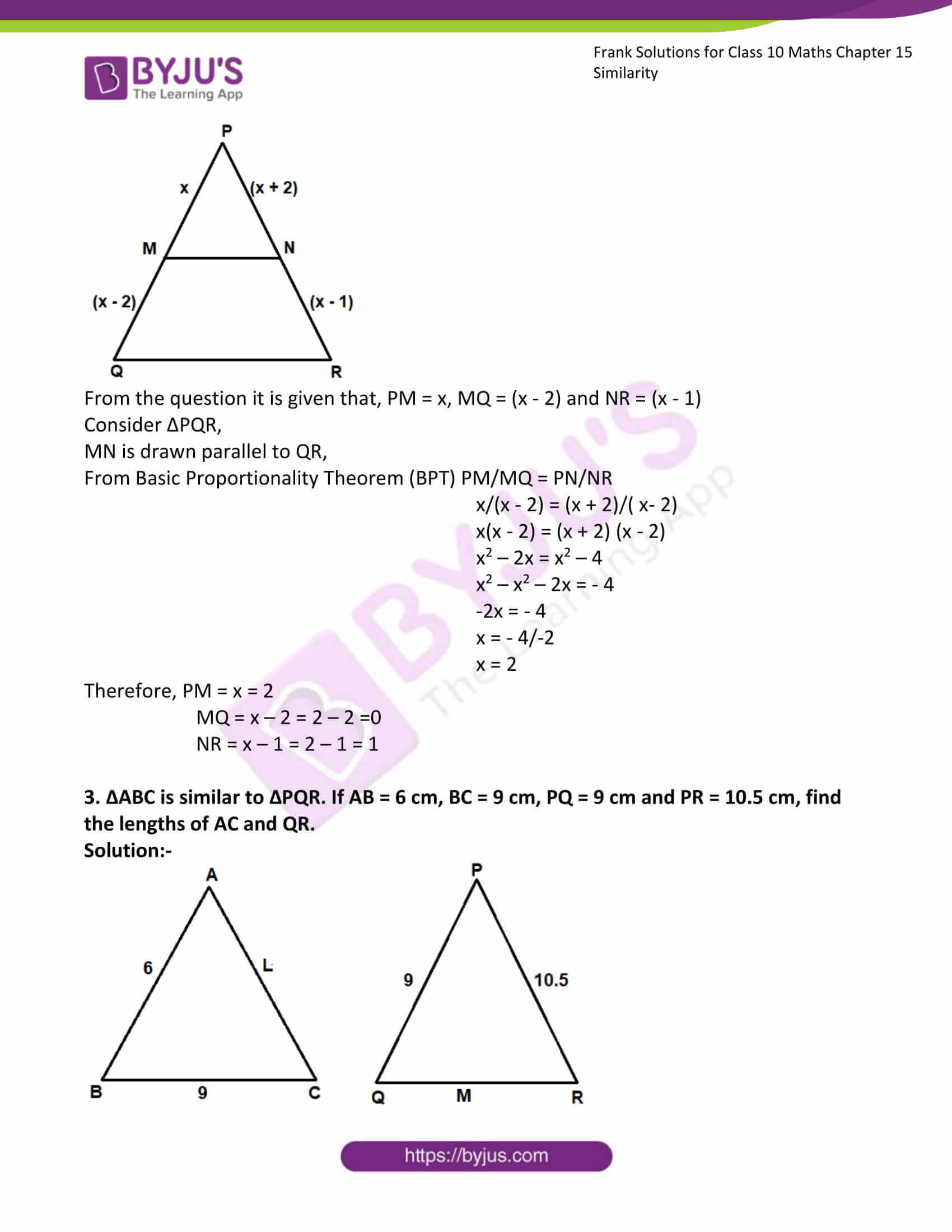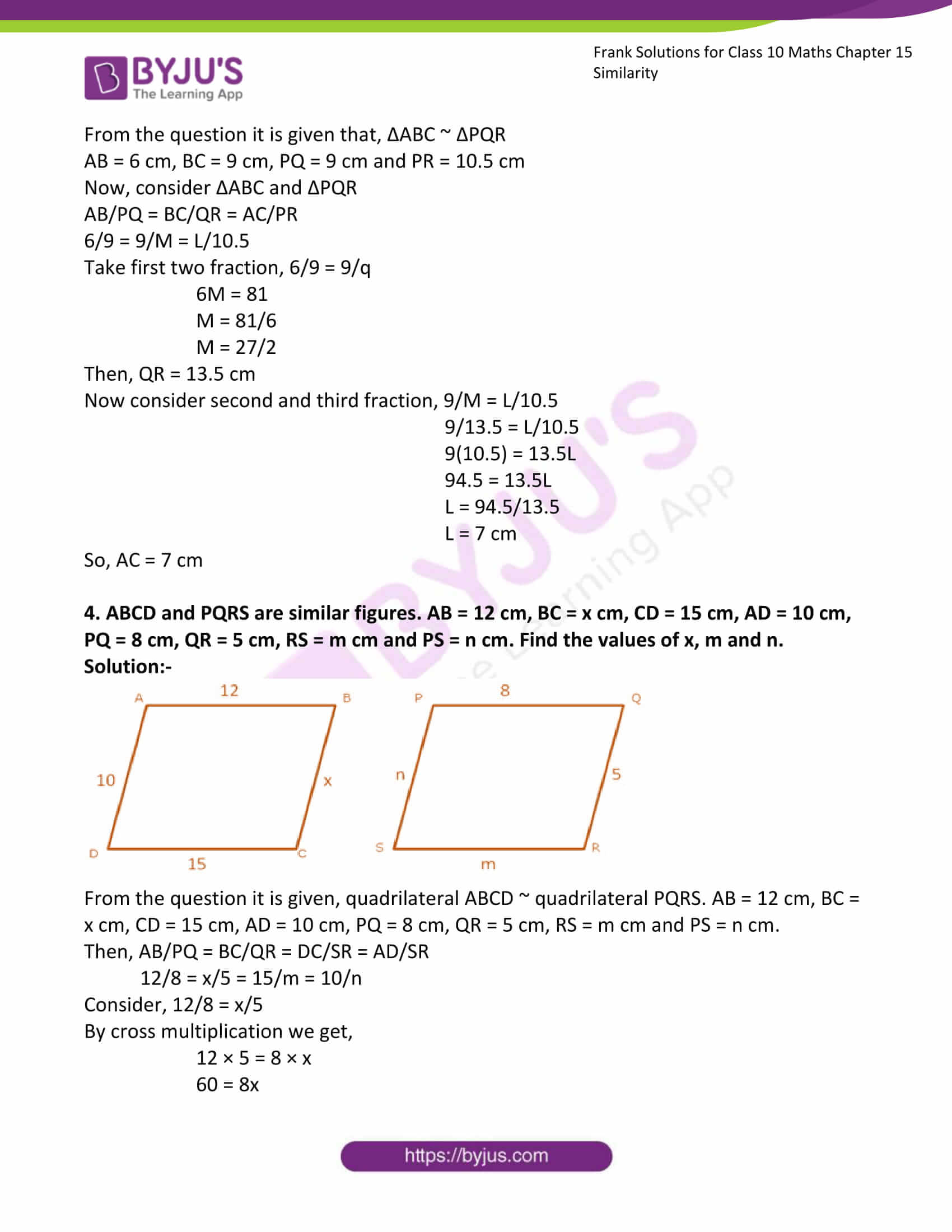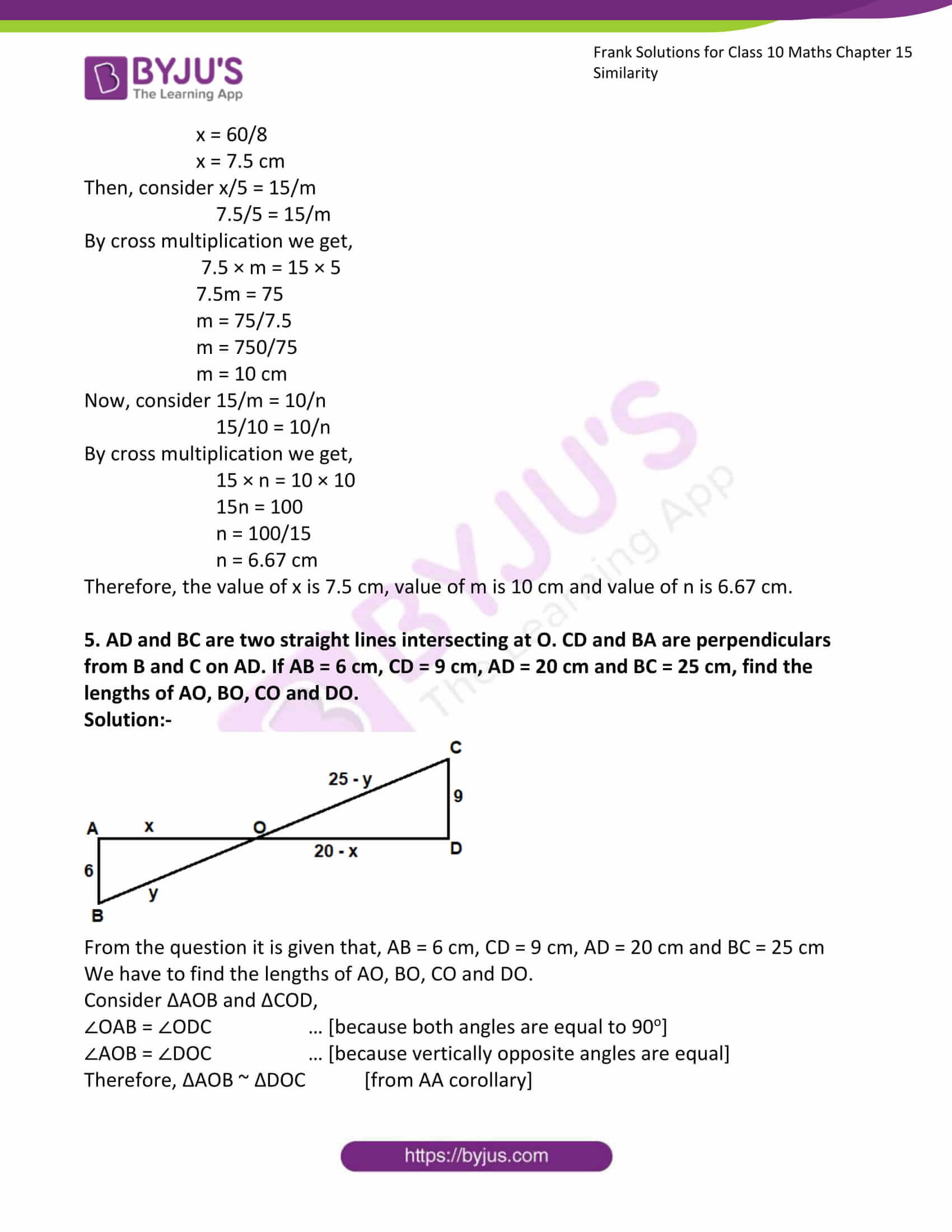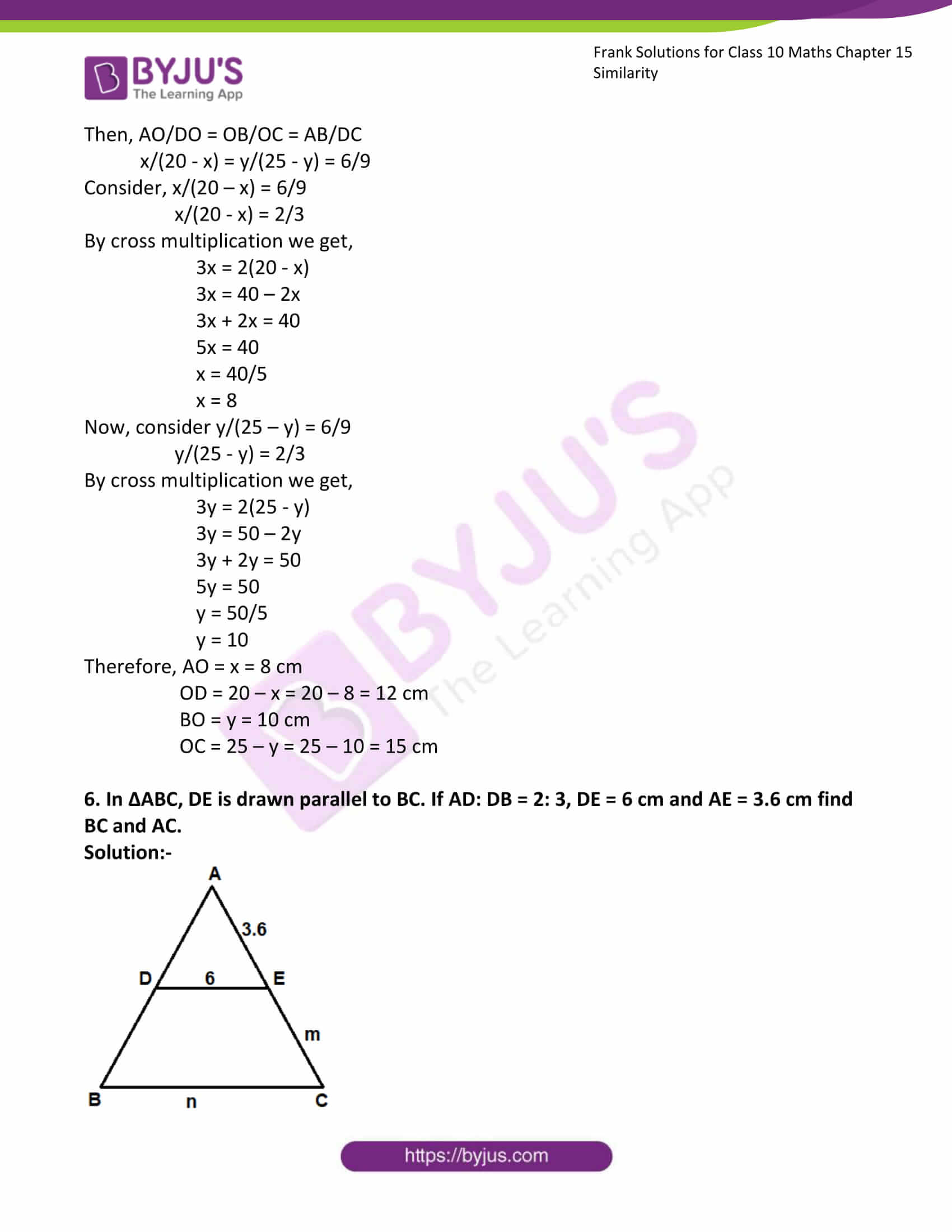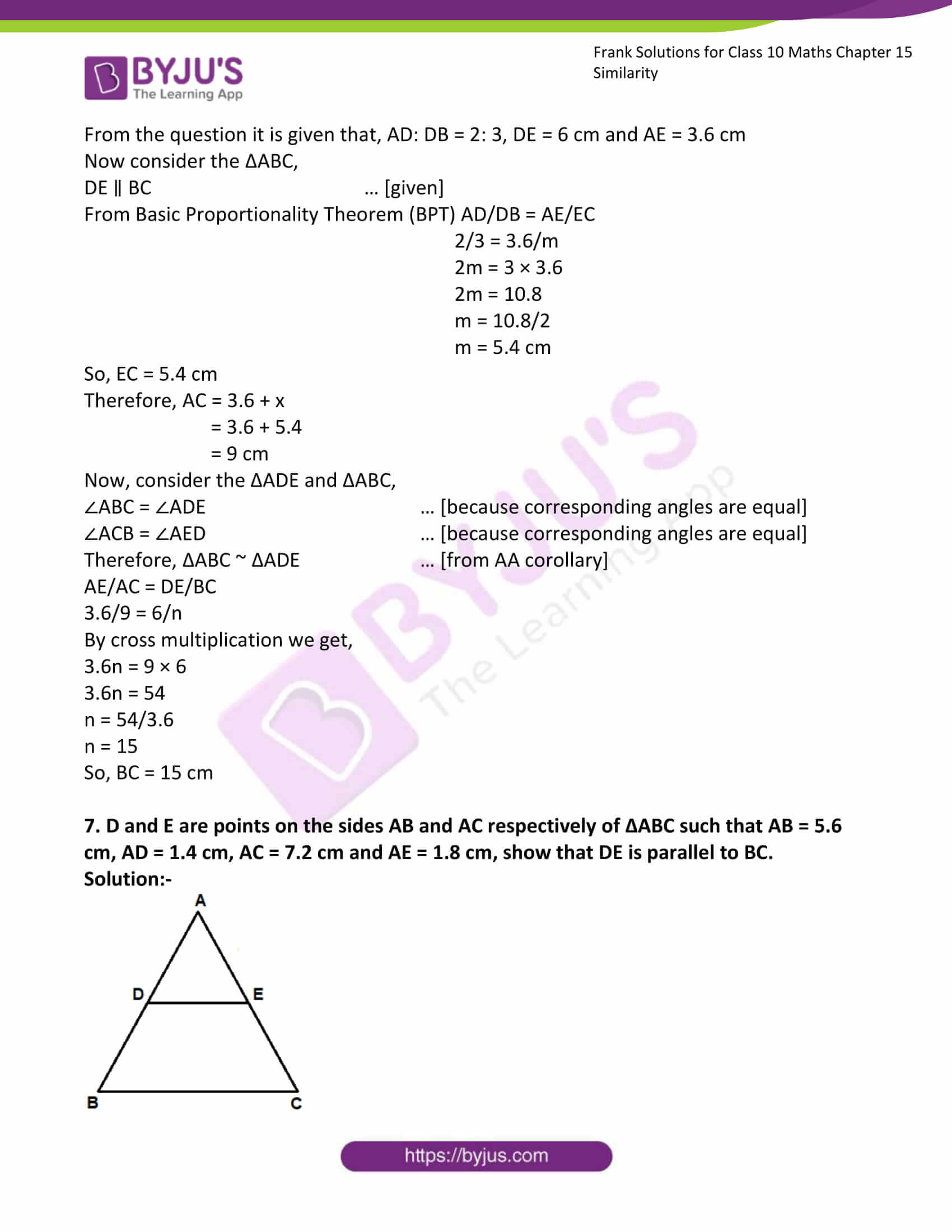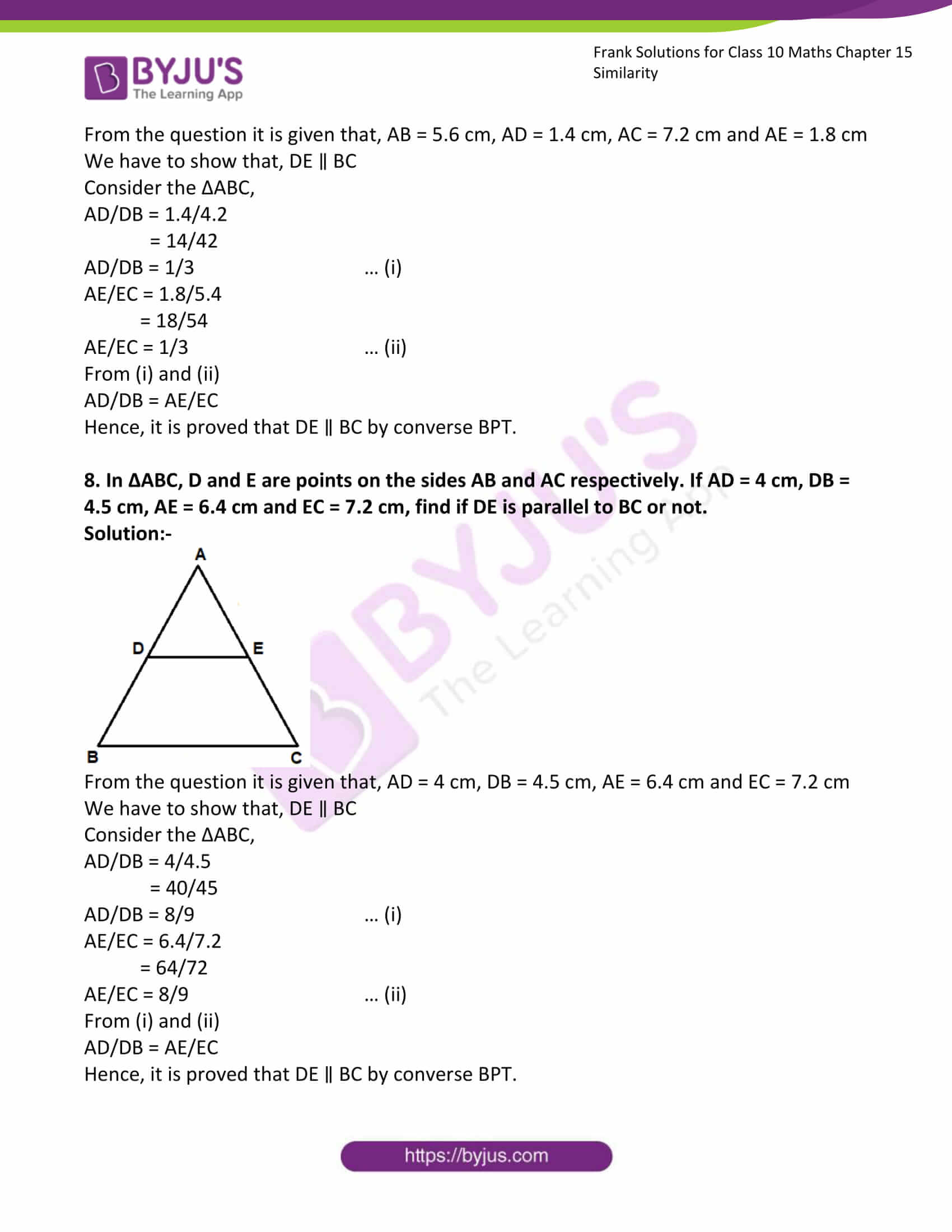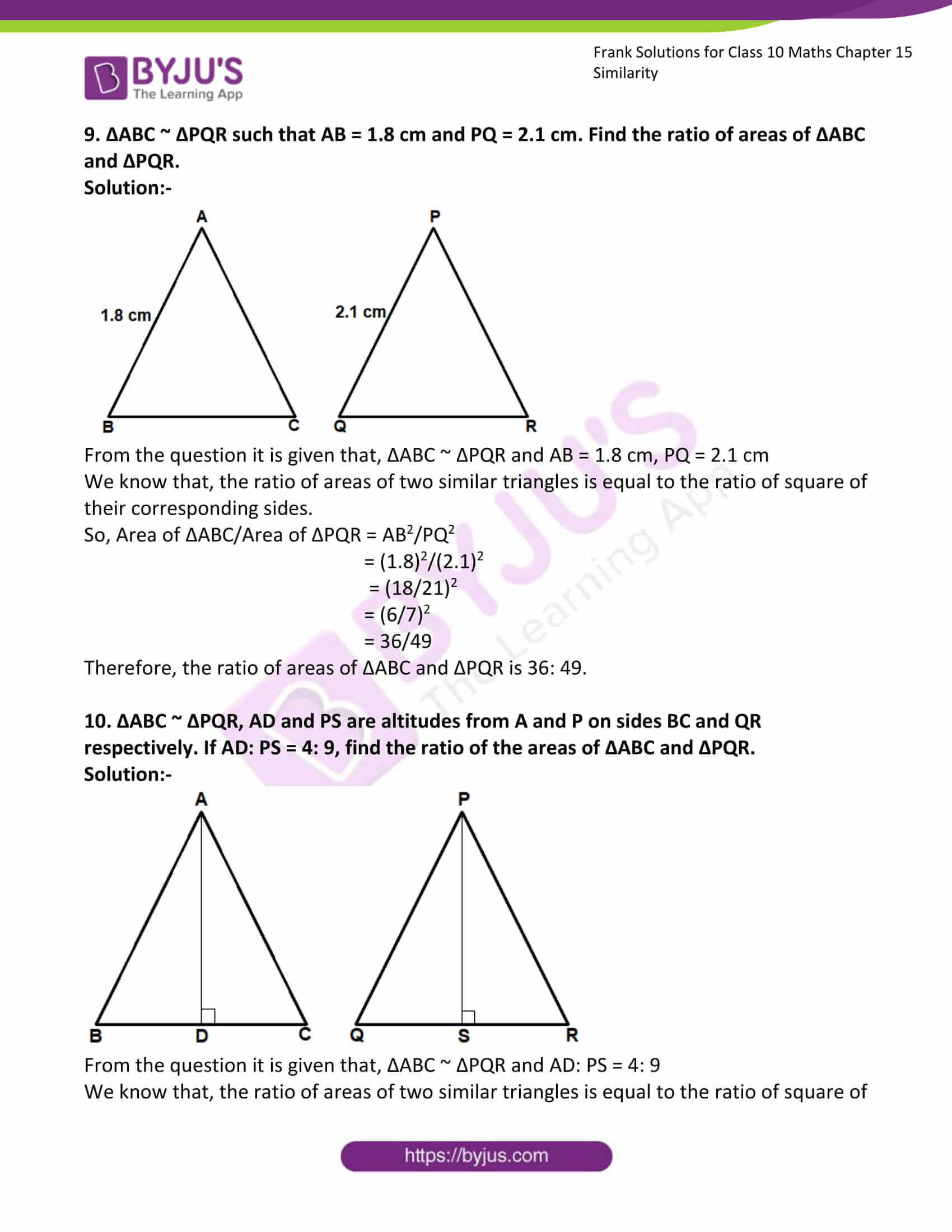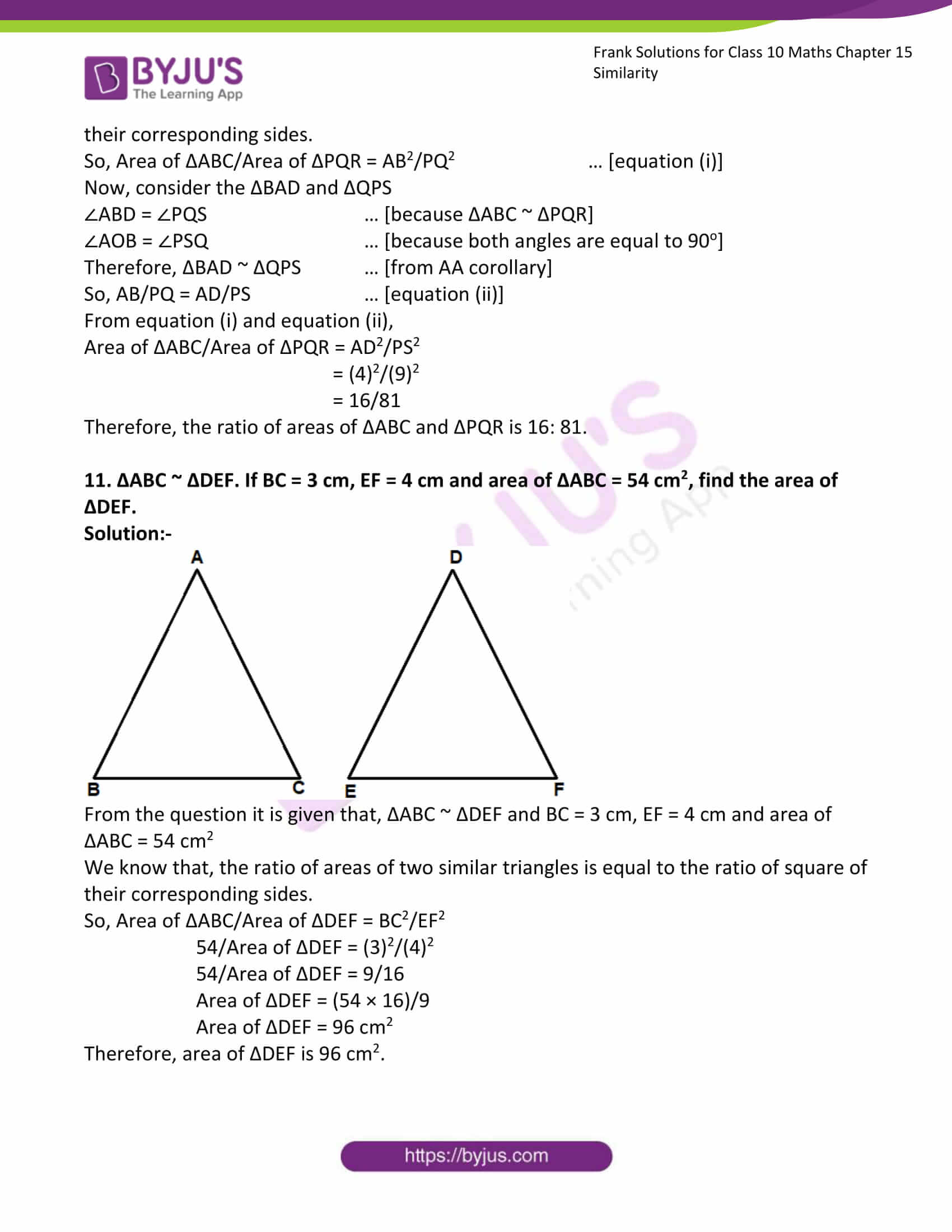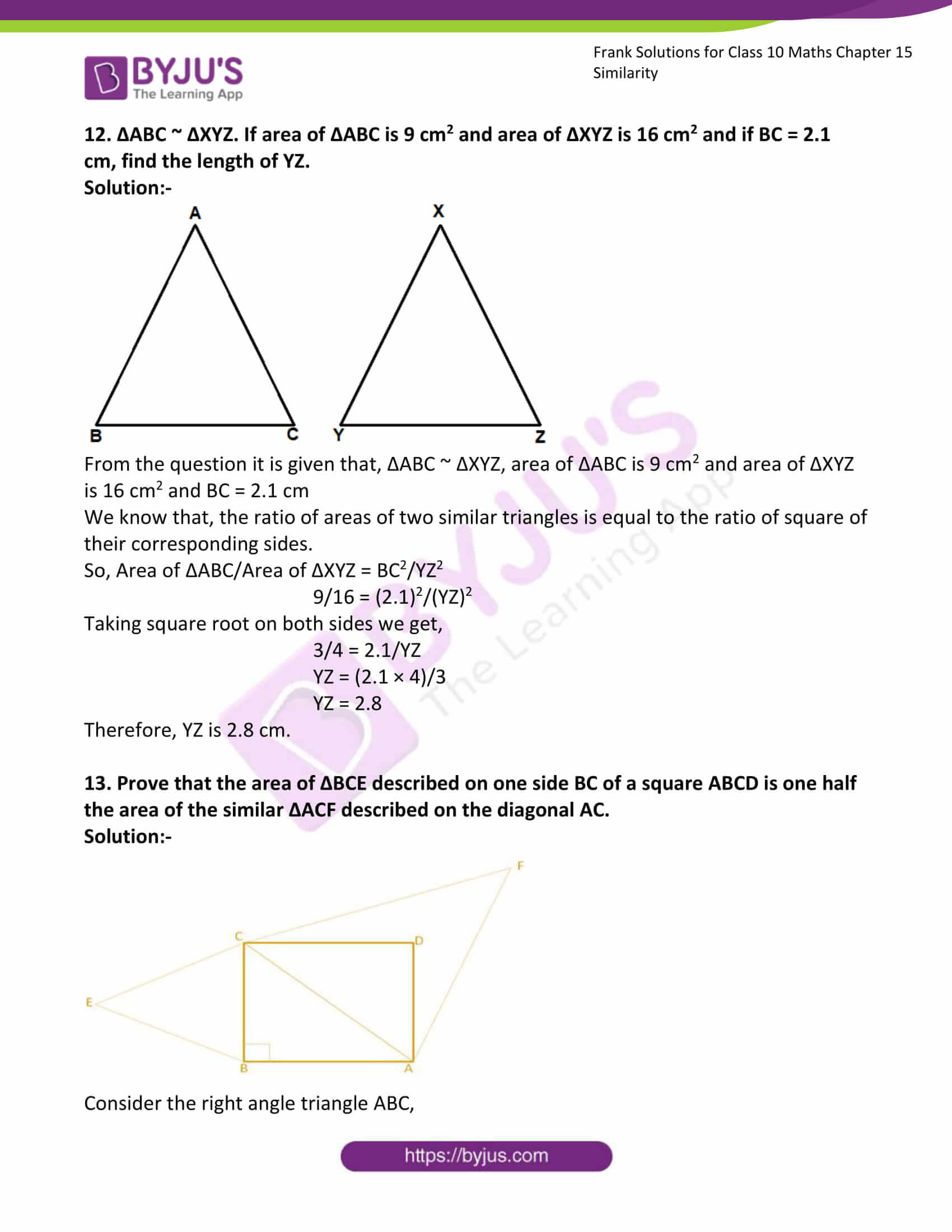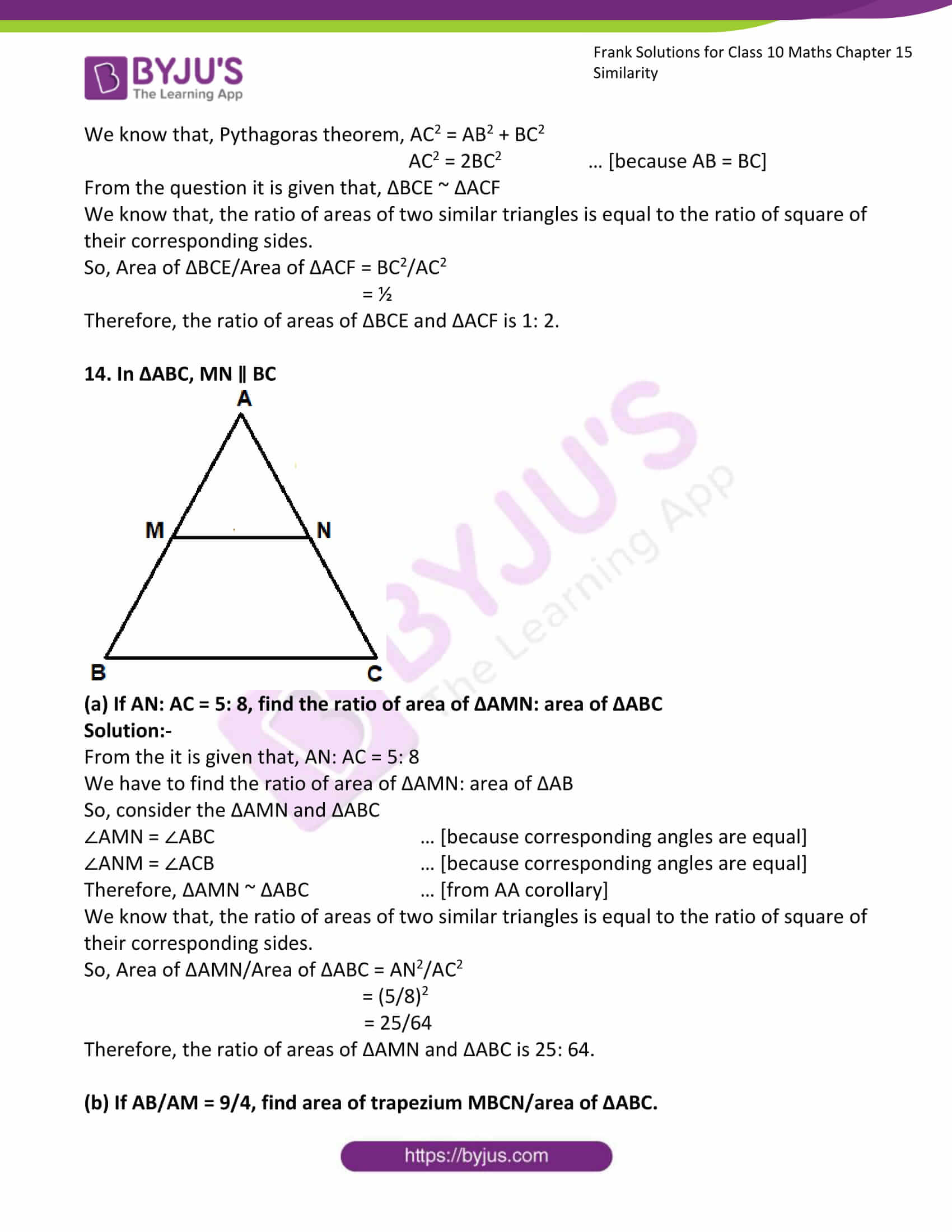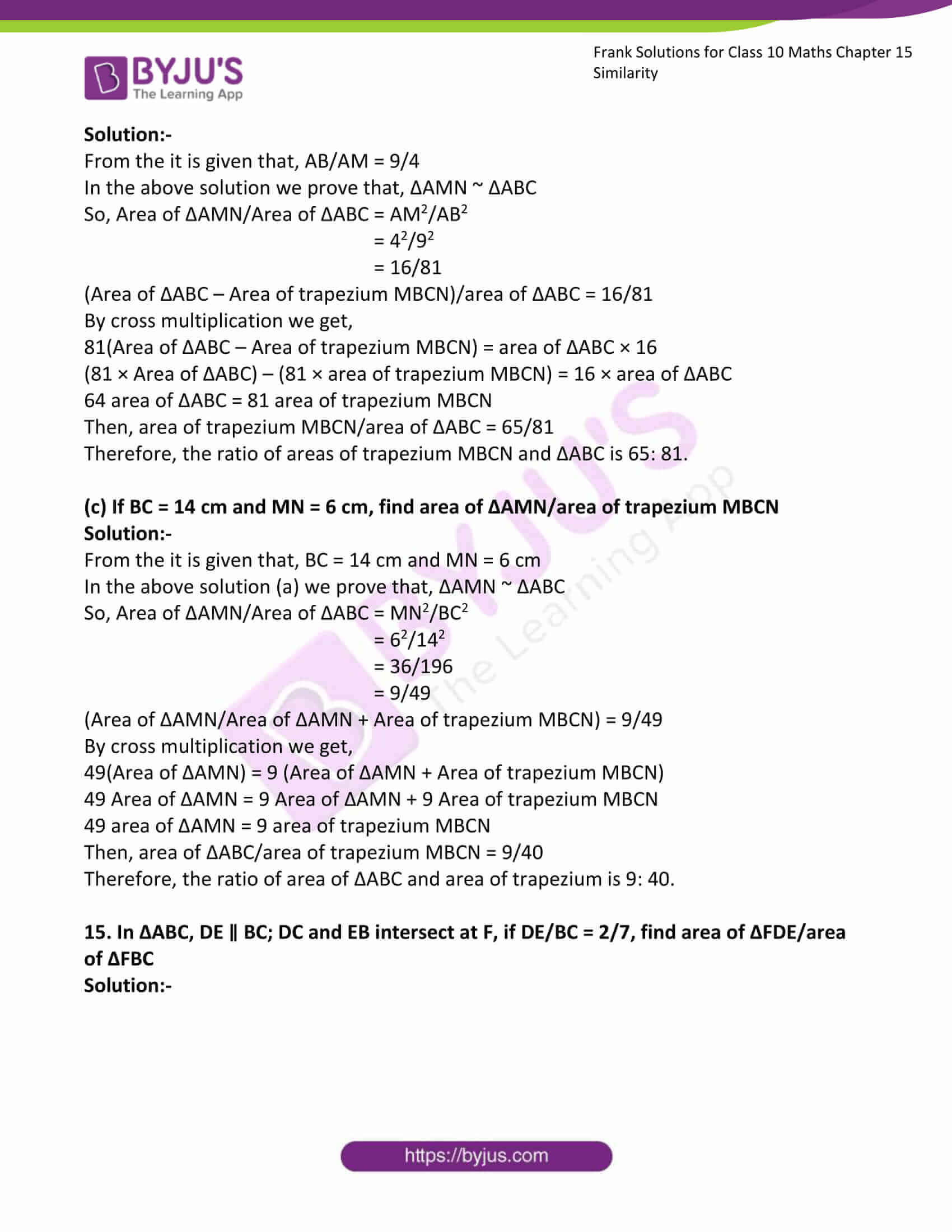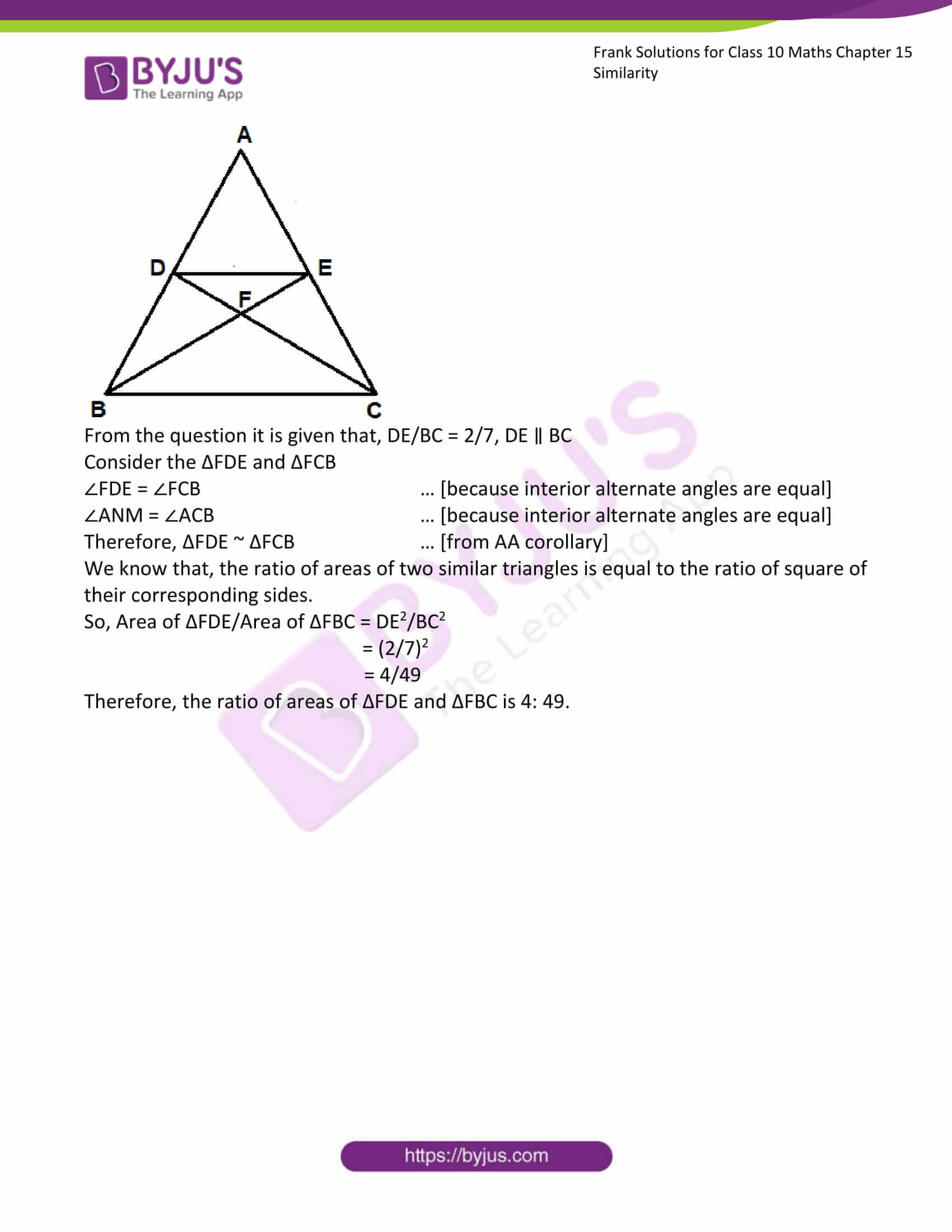### Access Answers to Frank Solutions for Class 10 Maths Chapter 15 Similarity

1. In ΔABC, DE is parallel to BC and AD/DB = 2/7. If AC = 5.6, find AE.

Solution:-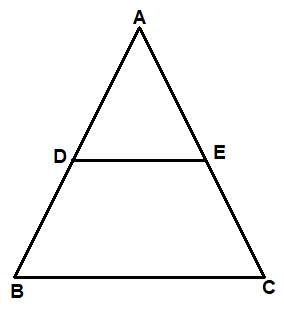From the question it is given that, AD/DB = 2/7 and AC = 5.6

Consider ΔABC,

DE is parallel to BC,

Let us assume AE = y

From Basic Proportionality Theorem (BPT) AD/DB = AE/EC

2/7 = y/(5.6 – y)

2(5.6 – y) = 7y

11.2 – 2y = 7y

11.2 = 7y + 2y

11.2 = 9y

y = 11.2/9

y = 1.24

Therefore, AE is 1.24

2. In ΔPQR, MN is drawn parallel to QR. If PM = x, MQ = (x – 2) and NR = (x – 1), find the value of x.

Solution:-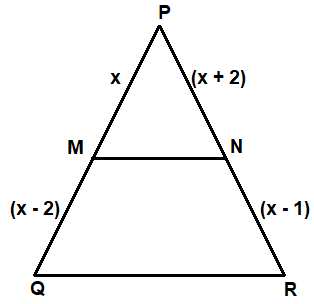From the question it is given that, PM = x, MQ = (x – 2) and NR = (x – 1)

Consider ΔPQR,

MN is drawn parallel to QR,

From Basic Proportionality Theorem (BPT) PM/MQ = PN/NR

x/(x – 2) = (x + 2)/( x- 2)

x(x – 2) = (x + 2) (x – 2)

x2 – 2x = x2 – 4

x2 – x2 – 2x = – 4

-2x = – 4

x = – 4/-2

x = 2

Therefore, PM = x = 2

MQ = x – 2 = 2 – 2 =0

NR = x – 1 = 2 – 1 = 1

3. ΔABC is similar to ΔPQR. If AB = 6 cm, BC = 9 cm, PQ = 9 cm and PR = 10.5 cm, find the lengths of AC and QR.

Solution:-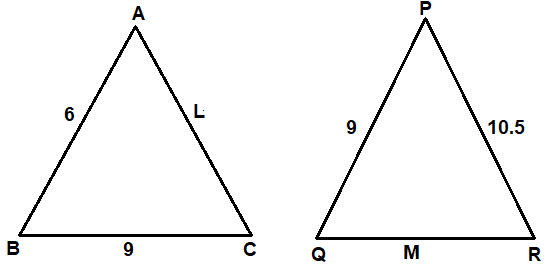From the question, it is given that, ΔABC ~ ΔPQR

AB = 6 cm, BC = 9 cm, PQ = 9 cm and PR = 10.5 cm

Now, consider ΔABC and ΔPQR

AB/PQ = BC/QR = AC/PR

6/9 = 9/M = L/10.5

Take first two fraction, 6/9 = 9/q

6M = 81

M = 81/6

M = 27/2

Then, QR = 13.5 cm

Now consider second and third fraction, 9/M = L/10.5

9/13.5 = L/10.5

9(10.5) = 13.5L

94.5 = 13.5L

L = 94.5/13.5

L = 7 cm

So, AC = 7 cm

4. ABCD and PQRS are similar figures. AB = 12 cm, BC = x cm, CD = 15 cm, AD = 10 cm, PQ = 8 cm, QR = 5 cm, RS = m cm and PS = n cm. Find the values of x, m and n.

Solution:-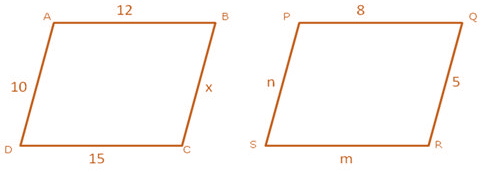From the question it is given, quadrilateral ABCD ~ quadrilateral PQRS. AB = 12 cm, BC = x cm, CD = 15 cm, AD = 10 cm, PQ = 8 cm, QR = 5 cm, RS = m cm and PS = n cm.

Then, AB/PQ = BC/QR = DC/SR = AD/SR

12/8 = x/5 = 15/m = 10/n

Consider, 12/8 = x/5

By cross multiplication, we get,

12 × 5 = 8 × x

60 = 8x

x = 60/8

x = 7.5 cm

Then, consider x/5 = 15/m

7.5/5 = 15/m

By cross multiplication, we get,

7.5 × m = 15 × 5

7.5m = 75

m = 75/7.5

m = 750/75

m = 10 cm

Now, consider 15/m = 10/n

15/10 = 10/n

By cross multiplication, we get,

15 × n = 10 × 10

15n = 100

n = 100/15

n = 6.67 cm

Therefore, the value of x is 7.5 cm, value of m is 10 cm and value of n is 6.67 cm.

5. AD and BC are two straight lines intersecting at O. CD and BA are perpendiculars from B and C on AD. If AB = 6 cm, CD = 9 cm, AD = 20 cm and BC = 25 cm, find the lengths of AO, BO, CO and DO.

Solution:-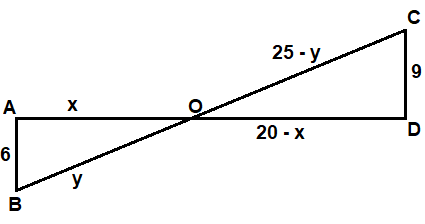From the question it is given that, AB = 6 cm, CD = 9 cm, AD = 20 cm and BC = 25 cm

We have to find the lengths of AO, BO, CO and DO.

Consider ΔAOB and ΔCOD,

∠OAB = ∠ODC … [because both angles are equal to 90o]

∠AOB = ∠DOC … [because vertically opposite angles are equal]

Therefore, ΔAOB ~ ΔDOC [from AA corollary]

Then, AO/DO = OB/OC = AB/DC

x/(20 – x) = y/(25 – y) = 6/9

Consider, x/(20 – x) = 6/9

x/(20 – x) = 2/3

By cross multiplication, we get,

3x = 2(20 – x)

3x = 40 – 2x

3x + 2x = 40

5x = 40

x = 40/5

x = 8

Now, consider y/(25 – y) = 6/9

y/(25 – y) = 2/3

By cross multiplication, we get,

3y = 2(25 – y)

3y = 50 – 2y

3y + 2y = 50

5y = 50

y = 50/5

y = 10

Therefore, AO = x = 8 cm

OD = 20 – x = 20 – 8 = 12 cm

BO = y = 10 cm

OC = 25 – y = 25 – 10 = 15 cm

6. In ΔABC, DE is drawn parallel to BC. If AD: DB = 2: 3, DE = 6 cm and AE = 3.6 cm find BC and AC.

Solution:-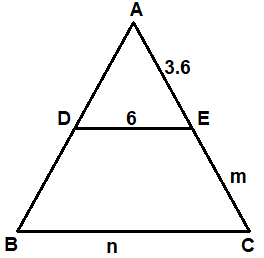From the question it is given that, AD: DB = 2: 3, DE = 6 cm and AE = 3.6 cm

Now consider the ΔABC,

DE ∥ BC … [given]

From Basic Proportionality Theorem (BPT) AD/DB = AE/EC

2/3 = 3.6/m

2m = 3 × 3.6

2m = 10.8

m = 10.8/2

m = 5.4 cm

So, EC = 5.4 cm

Therefore, AC = 3.6 + x

= 3.6 + 5.4

= 9 cm

Now, consider the ΔADE and ΔABC,

∠ABC = ∠ADE … [because corresponding angles are equal]

∠ACB = ∠AED … [because corresponding angles are equal]

Therefore, ΔABC ~ ΔADE … [from AA corollary]

AE/AC = DE/BC

3.6/9 = 6/n

By cross multiplication, we get,

3.6n = 9 × 6

3.6n = 54

n = 54/3.6

n = 15

So, BC = 15 cm

7. D and E are points on the sides AB and AC respectively of ΔABC such that AB = 5.6 cm, AD = 1.4 cm, AC = 7.2 cm and AE = 1.8 cm, show that DE is parallel to BC.

Solution:-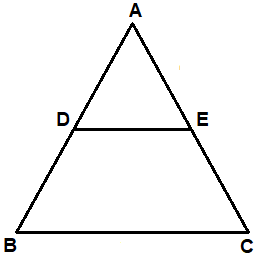From the question it is given that, AB = 5.6 cm, AD = 1.4 cm, AC = 7.2 cm and AE = 1.8 cm

We have to show that, DE ∥ BC

Consider the ΔABC,

= 14/42

AE/EC = 1.8/5.4

= 18/54

AE/EC = 1/3 … (ii)

From (i) and (ii)

Hence, it is proved that DE ∥ BC by converse BPT.

8. In ΔABC, D and E are points on the sides AB and AC respectively. If AD = 4 cm, DB = 4.5 cm, AE = 6.4 cm and EC = 7.2 cm, find if DE is parallel to BC or not.

Solution:-From the question it is given that, AD = 4 cm, DB = 4.5 cm, AE = 6.4 cm and EC = 7.2 cm

We have to show that, DE ∥ BC

Consider the ΔABC,

= 40/45

AE/EC = 6.4/7.2

= 64/72

AE/EC = 8/9 … (ii)

From (i) and (ii)

Hence, it is proved that DE ∥ BC by converse BPT.

9. ΔABC ~ ΔPQR such that AB = 1.8 cm and PQ = 2.1 cm. Find the ratio of areas of ΔABC and ΔPQR.

Solution:-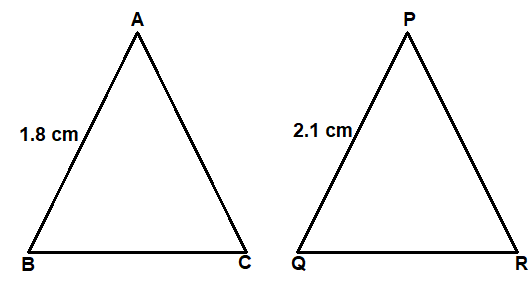From the question it is given that, ΔABC ~ ΔPQR and AB = 1.8 cm, PQ = 2.1 cm

We know that, the ratio of areas of two similar triangles is equal to the ratio of square of their corresponding sides.

So, Area of ΔABC/Area of ΔPQR = AB2/PQ2

= (1.8)2/(2.1)2

= (18/21)2

= (6/7)2

= 36/49

Therefore, the ratio of areas of ΔABC and ΔPQR is 36: 49.

10. ΔABC ~ ΔPQR, AD and PS are altitudes from A and P on sides BC and QR respectively. If AD: PS = 4: 9, find the ratio of the areas of ΔABC and ΔPQR.

Solution:-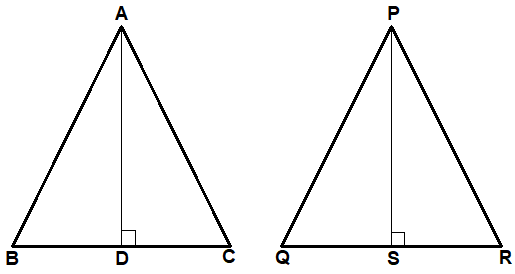From the question it is given that, ΔABC ~ ΔPQR and AD: PS = 4: 9

We know that, the ratio of areas of two similar triangles is equal to the ratio of square of their corresponding sides.

So, Area of ΔABC/Area of ΔPQR = AB2/PQ2 … [equation (i)]

Now, consider the ΔBAD and ΔQPS

∠ABD = ∠PQS … [because ΔABC ~ ΔPQR]

∠AOB = ∠PSQ … [because both angles are equal to 90o]

Therefore, ΔBAD ~ ΔQPS … [from AA corollary]

So, AB/PQ = AD/PS … [equation (ii)]

From equation (i) and equation (ii),

Area of ΔABC/Area of ΔPQR = AD2/PS2

= (4)2/(9)2

= 16/81

Therefore, the ratio of areas of ΔABC and ΔPQR is 16: 81.

11. ΔABC ~ ΔDEF. If BC = 3 cm, EF = 4 cm and area of ΔABC = 54 cm2, find the area of ΔDEF.

Solution:-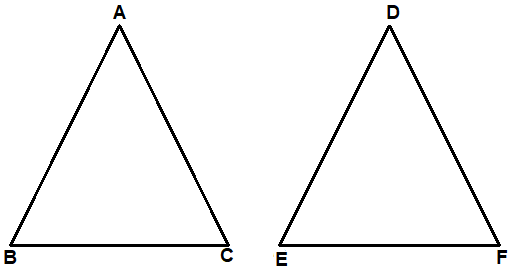From the question it is given that, ΔABC ~ ΔDEF and BC = 3 cm, EF = 4 cm and area of ΔABC = 54 cm2

We know that, the ratio of areas of two similar triangles is equal to the ratio of square of their corresponding sides.

So, Area of ΔABC/Area of ΔDEF = BC2/EF2

54/Area of ΔDEF = (3)2/(4)2

54/Area of ΔDEF = 9/16

Area of ΔDEF = (54 × 16)/9

Area of ΔDEF = 96 cm2

Therefore, area of ΔDEF is 96 cm2.

12. ΔABC ~ ΔXYZ. If area of ΔABC is 9 cm2 and area of ΔXYZ is 16 cm2 and if BC = 2.1 cm, find the length of YZ.

Solution:-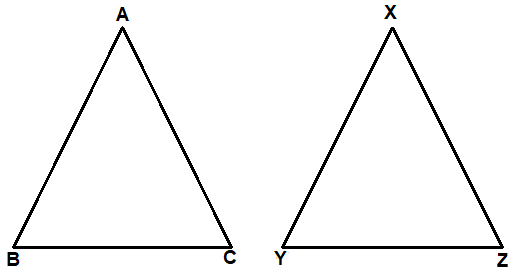From the question it is given that, ΔABC ~ ΔXYZ, area of ΔABC is 9 cm2 and area of ΔXYZ is 16 cm2 and BC = 2.1 cm

We know that, the ratio of areas of two similar triangles is equal to the ratio of square of their corresponding sides.

So, Area of ΔABC/Area of ΔXYZ = BC2/YZ2

9/16 = (2.1)2/(YZ)2

Taking square root on both sides we get,

3/4 = 2.1/YZ

YZ = (2.1 × 4)/3

YZ = 2.8

Therefore, YZ is 2.8 cm.

13. Prove that the area of ΔBCE described on one side BC of a square ABCD is one half the area of the similar ΔACF described on the diagonal AC.

Solution:-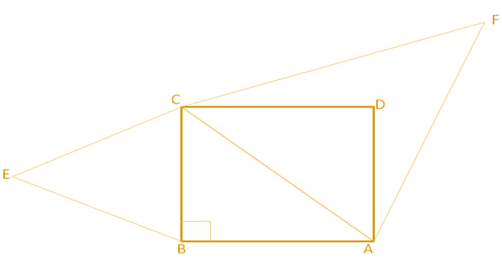Consider the right angle triangle ABC,

We know that, Pythagoras theorem, AC2 = AB2 + BC2

AC2 = 2BC2 … [because AB = BC]

From the question it is given that, ΔBCE ~ ΔACF

We know that, the ratio of areas of two similar triangles is equal to the ratio of square of their corresponding sides.

So, Area of ΔBCE/Area of ΔACF = BC2/AC2

= ½

Therefore, the ratio of areas of ΔBCE and ΔACF is 1: 2.

14. In ΔABC, MN ∥ BC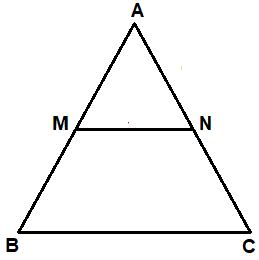(a) If AN: AC = 5: 8, find the ratio of area of ΔAMN: area of ΔABC

Solution:-

From the it is given that, AN: AC = 5: 8

We have to find the ratio of area of ΔAMN: area of ΔAB

So, consider the ΔAMN and ΔABC

∠AMN = ∠ABC … [because corresponding angles are equal]

∠ANM = ∠ACB … [because corresponding angles are equal]

Therefore, ΔAMN ~ ΔABC … [from AA corollary]

We know that, the ratio of areas of two similar triangles is equal to the ratio of square of their corresponding sides.

So, Area of ΔAMN/Area of ΔABC = AN2/AC2

= (5/8)2

= 25/64

Therefore, the ratio of areas of ΔAMN and ΔABC is 25: 64.

(b) If AB/AM = 9/4, find area of trapezium MBCN/area of ΔABC.

Solution:-

From the it is given that, AB/AM = 9/4

In the above solution we prove that, ΔAMN ~ ΔABC

So, Area of ΔAMN/Area of ΔABC = AM2/AB2

= 42/92

= 16/81

(Area of ΔABC – Area of trapezium MBCN)/area of ΔABC = 16/81

By cross multiplication we get,

81(Area of ΔABC – Area of trapezium MBCN) = area of ΔABC × 16

(81 × Area of ΔABC) – (81 × area of trapezium MBCN) = 16 × area of ΔABC

64 area of ΔABC = 81 area of trapezium MBCN

Then, area of trapezium MBCN/area of ΔABC = 65/81

Therefore, the ratio of areas of trapezium MBCN and ΔABC is 65: 81.

(c) If BC = 14 cm and MN = 6 cm, find area of ΔAMN/area of trapezium MBCN

Solution:-

From the it is given that, BC = 14 cm and MN = 6 cm

In the above solution (a) we prove that, ΔAMN ~ ΔABC

So, Area of ΔAMN/Area of ΔABC = MN2/BC2

= 62/142

= 36/196

= 9/49

(Area of ΔAMN/Area of ΔAMN + Area of trapezium MBCN) = 9/49

By cross multiplication we get,

49(Area of ΔAMN) = 9 (Area of ΔAMN + Area of trapezium MBCN)

49 Area of ΔAMN = 9 Area of ΔAMN + 9 Area of trapezium MBCN

49 area of ΔAMN = 9 area of trapezium MBCN

Then, area of ΔABC/area of trapezium MBCN = 9/40

Therefore, the ratio of area of ΔABC and area of trapezium is 9: 40.

15. In ΔABC, DE ∥ BC; DC and EB intersect at F, if DE/BC = 2/7, find area of ΔFDE/area of ΔFBC

Solution:-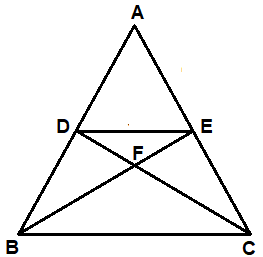From the question it is given that, DE/BC = 2/7, DE ∥ BC

Consider the ΔFDE and ΔFCB

∠FDE = ∠FCB … [because interior alternate angles are equal]

∠ANM = ∠ACB … [because interior alternate angles are equal]

Therefore, ΔFDE ~ ΔFCB … [from AA corollary]

We know that, the ratio of areas of two similar triangles is equal to the ratio of square of their corresponding sides.

So, Area of ΔFDE/Area of ΔFBC = DE2/BC2

= (2/7)2

= 4/49

Therefore, the ratio of areas of ΔFDE and ΔFBC is 4: 49.